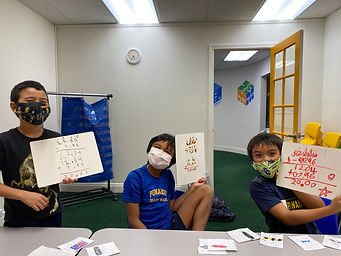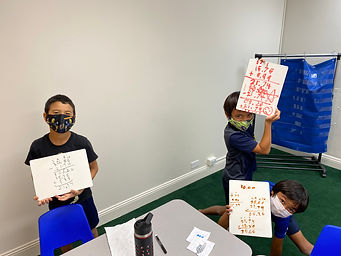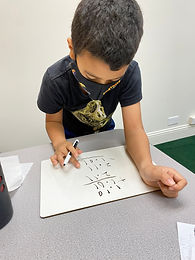## Ms. Amanda

### Target 1​

###### Lesson Type:

Continuation

Number Operation

:

Computation

Multiply within 100.

###### 1:

Understand that multiplying numbers makes numbers larger.

###### 2:

Understand that multiplication represents the combination of equal groups of objects.

###### 3:

Understand that multiplication can be represented with arrays, repeated addition, and skip counting.

3rd

###### Vocabulary:

Activities:

- Students used different multiplication strategies to solve multiplication facts.

- Students played multiplication bingo to practice multiplication facts.### Home Exploration

###### Guiding Questions:## Absent Students:

### Target 2

:

###### 1:

Understand that when subtracting a multi-digit number, each place-value is being subtracted individually to create the new number (ones are subtracted from ones, tens are subtracted from tens, etc.)

###### 2:

Understand how to regroup based on place-value understandings (i.e., ten ones can be composed into one ten – ten tens can be composed into one hundred).

###### 3:

Understand that subtraction equations can be written both vertically and horizontally.

###### 4:

Understand that the same process is utilized to subtract three or more whole numbers that is used to subtract only two numbers.

3rd

###### Vocabulary:

Subtract, Borrow, Regroup

Activities:

- Students solved subtraction problems with up to 4 digits and with regrouping.

- Students solved subtraction problems with money.

- Students "bought" items from each other, set up the subtraction problem to determine how much they would receive, and practiced subtracting to find the change.

- Students checked their work using addition.### Home Exploration

John buys a t-shirt for \$13.49. He pays with a \$20.00 bill. How much change will John get?

\$6.51

###### Guiding Questions:### Target 3

:

###### 1:

Solve problems that have more than one operation.

###### Vocabulary:

Activities:

- Students "bought" two items from each other and figured how much change they would get.

- Students set up the addition problem first, then set up the subtraction problem to determine how much change they would receive.

- Students checked their work using addition.### Home Exploration